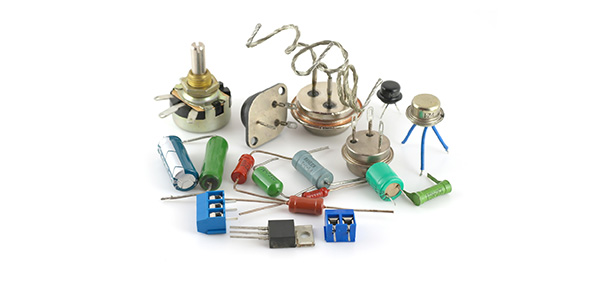# Short-circuits

8 Questions | Total Attempts: 80Settings.

Related Topics
• 1.
How does a circuit work?
• A.

Electrons circle around the circuit, speeding up each time, as long as the circuit is open.

• B.

Electrons circle around the circuit, speeding up each time, as long as the circuit is closed.

• C.

Electrons circle around the circuit, slowing down each time, as long as the circuit is closed.

• D.

Electrons go up and down, creating electrical power.

• 2.
The job of a resistor is to act as a roadblock and lower the level of voltage. This stops the circuit from burning up.
• A.

True

• B.

False

• 3.
Is it true an IC chip, a transistor and a relay are all types of resistors?
• A.

True

• B.

False

• 4.
What do the four coloured stripes on a resistor stand for (in order)?
• A.

Digit 1, Digit 2, the level of tolerance, and the multiplier

• B.

Digit 1, Digit 2, Digit 3, and the multiplier

• C.

Digit 1, Digit 2, Digit 3, and the level of tolerance

• D.

Digit 1, Digit 2, the multiplier, and the level of tolerance

• 5.
What is the p-n junction?
• A.

A boundary between two semi-conductive materials in the same shell. This is used to light an LED (Light Emitting Diode)

• B.

A junction where two pieces of wire meet

• C.

The terminal clips (connecting pieces)

• D.

A junction between two metals which let voltage flow through them

• 6.
A resistor is made from carbon or other semi-conductive materials.
• A.

True

• B.

False

• 7.
The three prongs on a transistor are called the base, the collector and the emitter. A transistor is "polarised", meaning that it can only be fitted one way round
• A.

True

• B.

False

• 8.
The resistance of a resistor is measured in ohms Ω
• A.

True

• B.

False